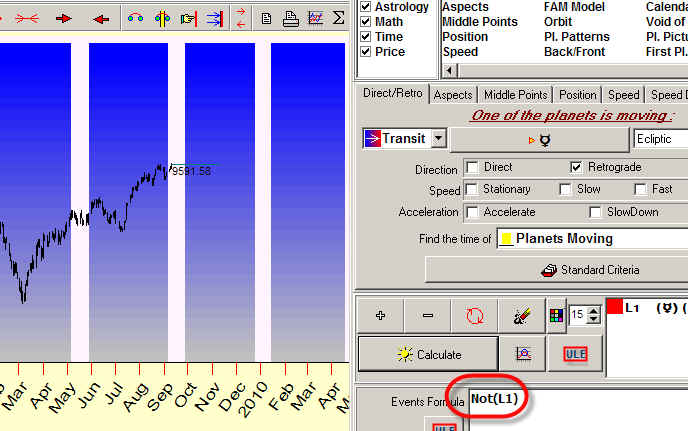Functions in ULE

Here I will show several examples of creating ULE formulas with Timing Solution software. (I will not explain what ULE is or how it works. You are supposed to study the related material on this website.)

Look at the screenshot below. Here you can see four ULE terms created: L1 - Sun declination, L2 - Mercury declination, L3 - Venus declination, and L4 - Mars declination: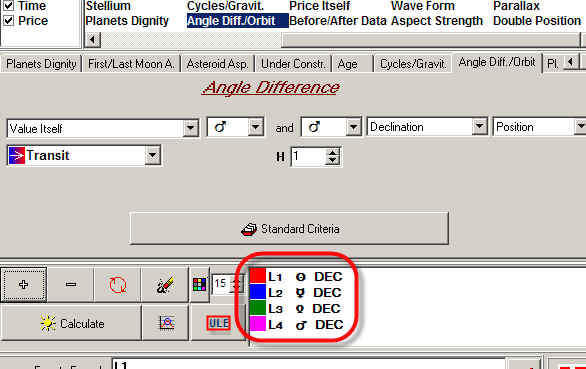It is not a formula yet, these are just 4 terms to start with.

If you need to display the summary declination of these four objects, type L1+L2+L3+L4 formula.

And now we will look into what can be done with this formula.

First of all, see this variation of this formula Sun+ Mercury-Venus-Mars declination. Remember that we are not discussing what this variation may represent or how it has been obtained. Instead, we can show how this formula "sounds" in time: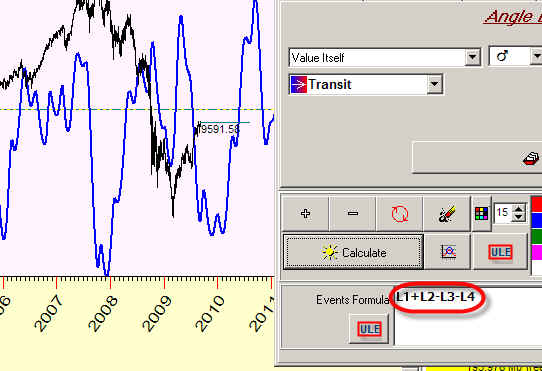There are several functions there created for your convenience.

If you need, you can calculate the sinus wave of this summary declination. Change the previous formula this way (type "Sin" before the formula: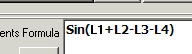Abs function allows to display the absolute value of this summary declination: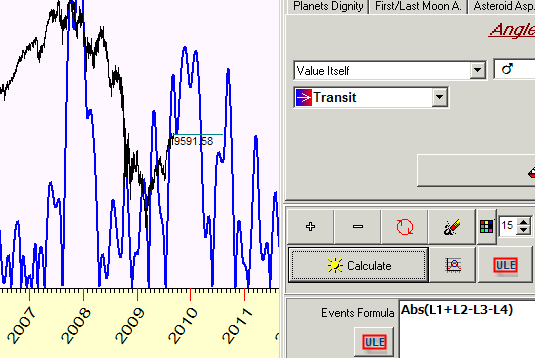Remember that this value is always positive. For example Abs(+20)=+20 and Abs(-20)=+20

Sqrt function calculates the square root: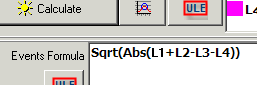You can modify the initial formula like this: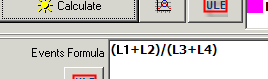This is (Sun + Mercury declination) divided by (Venus + Mars declination).

Positive function shows the positive part of diagram, like this (the negative part of this diagram is excluded):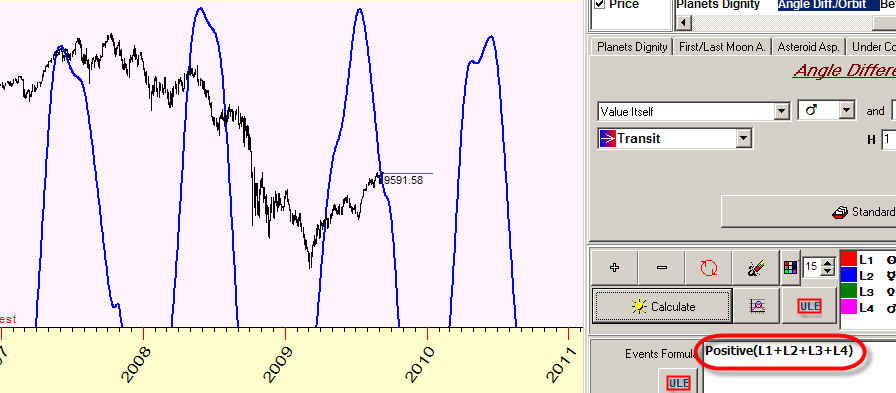Range function allows to perform filtering.

In this example, the program displays the periods when the summary declination varies from (-10) to (+10), the range is 10: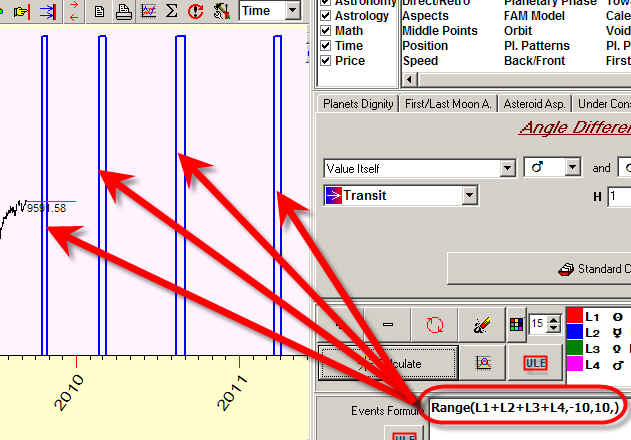Lets consider one more example. Let us take some other event, not declination. See the periods of retrograde Mercury: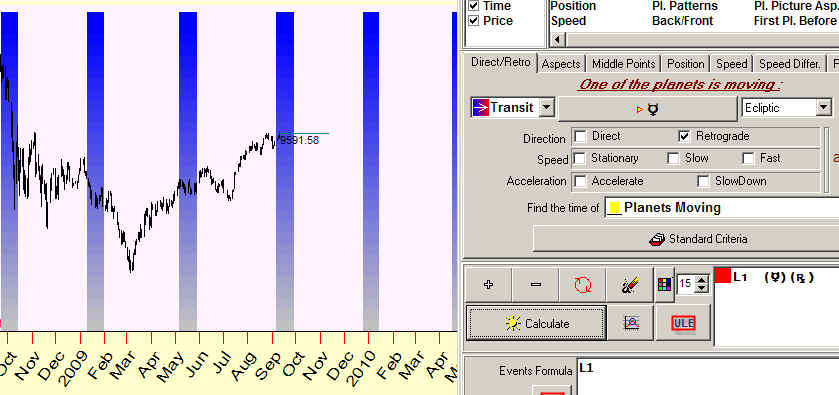Not function shows the periods when Mercury is NOT retrograde: